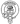# JavaScript default function parameters

June 26, 2020

In JavaScript, function parameters default to `undefined`. Often though we find ourselves wanting to instead provide a default value for the parameter.

Recently I came across a snippet from a project written long before we had all the fancy bells and whistles of ES6 that looked a little bit like this, I’ve stripped out any complexity because it’s not really relevant here.

``````function calculatePrice(price, quantity, modifier) {
modifier = modifier || 10

// calculate the price and return it
}``````

At first glance it seems to be simple enough it’s a function for calculating the price of something and accepts a price, quantity a modifier param which defaults to 10.

But if you’ve got a keen eye you might be able to spot the glaring issue with this code that could lead to items being priced completely wrong! Let’s take a look:

``````calculatePrice(25, 3) // modifier = 10
calculatePrice(12, 4, 15) // modifier = 15
calculatePrice(10, 1, 0) // modifier = 10``````

You’ll notice that in the last call despite passing `0` as the modifier to the function it’s still using the default value. That’s because in JavaScript the number `0` is falsy, a fancy way of saying it evaluates to false. This causes us to fall back on the default value. So let’s fix it first without the help of ES6:

``````function calculatePrice(price, quantity, modifier) {
if (typeof modifier === 'undefined') {
modifier = 10
}

// calculate the price and return it
}``````

By checking the type of the parameter is `undefined` we can ensure that we only default when no parameter is passed to the function.

``````calculatePrice(25, 3) // modifier = 10
calculatePrice(12, 4, 15) // modifier = 15
calculatePrice(10, 1, 0) // modifier = 0``````

Now when we pass in 0 as the modifier it doesn’t default. We can actually further improve our code by converting it to modern JavaScript. Default parameters are such a common use case that with ES6 there’s a far easier way of dealing with them.

``````function calculatePrice(price, quantity, modifier = 10) {
// calculate the price and return it
}``````

``````calculatePrice(25, 3) // modifier = 10
calculatePrice(12, 4, 15) // modifier = 15
calculatePrice(10, 1, 0) // modifier = 0``````

Less code, more readable and the same result.Written by Fraser Hamilton a full stack developer based out of Edinburgh, Scotland.